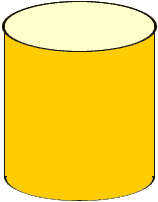Quandaries and Queries Question: where can you find how to get volume of a tube: 3 in across x 3 inch high 5 in across x 5 inch high same formula for both. ONE will get the other. thanks,mike Hi Mike, You didn't say so but I assume that the cross section of your tube is a circle.The volume of a tube is the area of the base times the height, so if the cross section is a circle you need to know how to find the area of a circle. The area of a circle is pi times the square of the radius, and henve the volume of a tube is volume =r2 h where r is the radius and h is the height. In your first tube the radius is 1.5 inches and the hieght is 3 inches so volume =r2 h = 3.14161.523 = 21.21 cubic inches Penny Go to Math Central